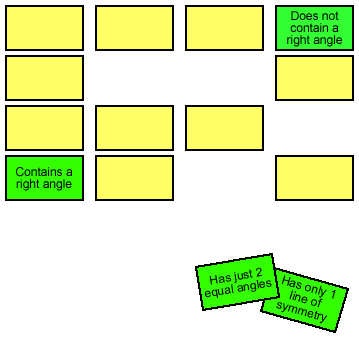#### You may also like### Kissing Triangles

Determine the total shaded area of the 'kissing triangles'.### Isosceles

Prove that a triangle with sides of length 5, 5 and 6 has the same area as a triangle with sides of length 5, 5 and 8. Find other pairs of non-congruent isosceles triangles which have equal areas.### Trice

ABCDEFGH is a 3 by 3 by 3 cube. Point P is 1/3 along AB (that is AP : PB = 1 : 2), point Q is 1/3 along GH and point R is 1/3 along ED. What is the area of the triangle PQR?

# Shapely Pairs

##### Age 11 to 14Challenge Level

Shapely Pairs printable sheet - triangle cards
Shapely Pairs printable sheet - quadrilateral cards

For this challenge, you will need to print out a set of triangle cards.

The first part is a game for two or more players; then there are some questions you can think about.

This type of game is played with lots of different sorts of cards; you might have heard it called Pairs, or Pelmanism. To play this version of the game, shuffle the cards and then lay them face down on the table, arranged in rows. Players take it in turns to turn over two cards. If the player can draw a triangle with the two properties shown, then s/he takes the cards. If not, once all the players have had a chance to look at the two cards and see where they belong, the cards are turned back over. Of course it will help you if you can remember where the cards are! The game finishes when no matter which two cards are turned over, there is no triangle with both of those properties. The winner is the person with the most cards at the end of the game. Good luck!You could also play the game using the quadrilateral cards.

Now here are some questions to get you thinking. Use the triangle cards for these.

Suppose instead of having the cards face down we have them all face up. If it's your turn first, how many possible pairs of cards are there that you could choose and win (that is, in how many ways could you choose a pair so that there is a triangle with both the properties)? Can you list all the possible pairs?

At the end of the game, you might be left with some cards that can't be paired up. What's the largest number you could be left with like this? What's the smallest? Give examples for each.

Now suppose that you want to make a pile of cards so that no matter which two you pick, you can always draw a triangle with both those properties. How big could the pile be? Can you give an example?This problem is based on the Triangle Property Game from "Geometry Games", a photocopiable resource produced by Gillian Hatch and available from the Association of Teachers of Mathematics